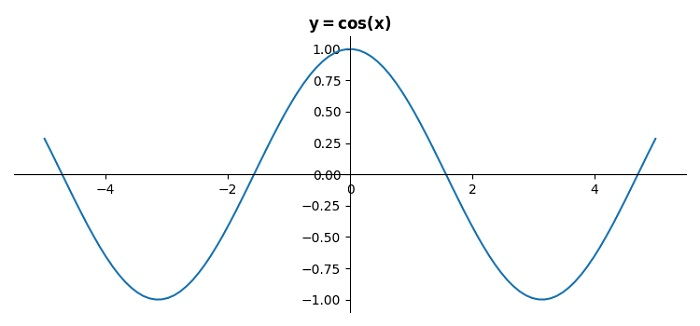# How to put the Origin at the center of the cos curve in a figure in Python Matplotlib?

To put the Origin at the center of the cos curve in a figure, we can take the following steps −

• Set the figure size and adjust the padding between and around the subplots.

• Create x and y data points using numpy.

• Set the position of the axes using spines, top, left, right and bottom.

• Plot x and y data points using plot() method.

• Set the title of the plot.

• To display the figure, use show() method.

## Example

import numpy as np
from matplotlib import pyplot as plt
plt.rcParams["figure.figsize"] = [7.50, 3.50]
plt.rcParams["figure.autolayout"] = True

x = np.linspace(-5, 5, 100)
y = np.cos(x)

ax = plt.gca()
ax.spines['top'].set_color('none')
ax.spines['left'].set_position('zero')
ax.spines['right'].set_color('none')
ax.spines['bottom'].set_position('zero')

plt.plot(x, y)
plt.title("$\bf{y=cos(x)}$")

plt.show()

## Output